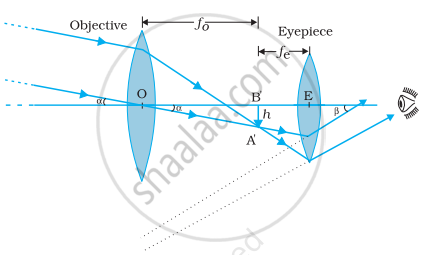# At What Distance Should the Lens Be Held from the Figure in Exercise 9.29 in Order to View the Squares Distinctly with the Maximum Possible Magnifying Power? - Physics

Numerical

(a) At what distance should the lens be held from the figure in order to view the squares distinctly with the maximum possible magnifying power?

(b) What is the magnification in this case?

(c) Is the magnification equal to the magnifying power in this case? Explain.#### Solution

(a) The maximum possible magnification is obtained when the image is formed at the near point (d = 25 cm).

Image distance, v = −d = −25 cm

Focal length, f = 10 cm

Object distance = u

According to the lens formula, we have:

1/"f" = 1/"v" - 1/"u"

1/"u" = 1/"v" - 1/"f"

= 1/(-25) - 1/10

= (-2-5)/50

= - 7/50

∴ u  = 50/7 = −7.14 cm

Hence, to view the squares distinctly, the lens should be kept 7.14 cm away from them.

(b) Magnification = |"v"/"u"| = 25/(50/7) = 3.5

(c) Magnifying power = "d"/"u" = 25/(50/7) = 3.5

Since the image is formed at the near point (25 cm), the magnifying power is equal to the magnitude of magnification.

Concept: Power of a Lens
Is there an error in this question or solution?

#### APPEARS IN

NCERT Physics Part 1 and 2 Class 12
Chapter 9 Ray Optics and Optical Instruments
Exercise | Q 9.24 | Page 347
NCERT Class 12 Physics Textbook
Chapter 9 Ray Optics and Optical Instruments
Exercise | Q 30 | Page 348

Share